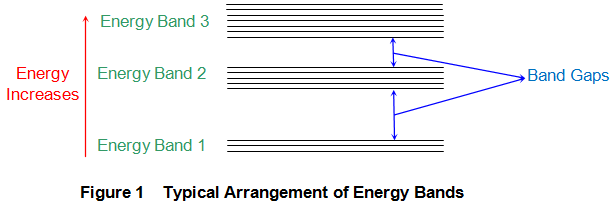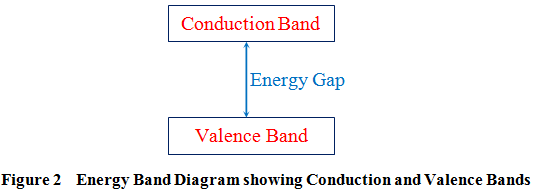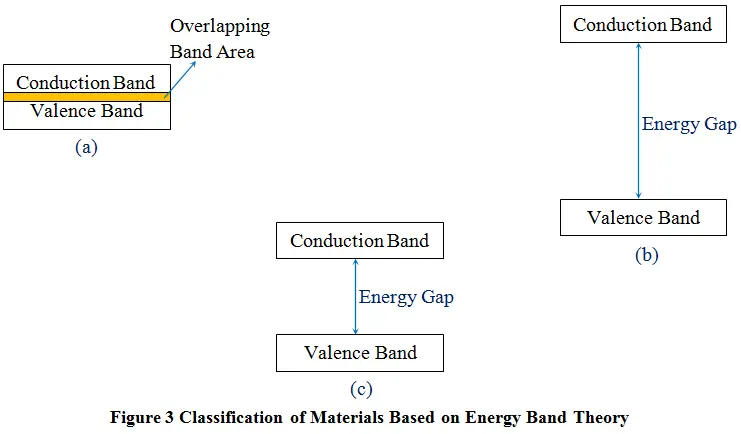# Energy Bands in Crystals

As per Neil Bohr’s theory of atomic structure, all the atoms are found to have discrete energy levels around their central nucleus (more on this can be found in the article “Atomic Energy Levels”). Now consider the case wherein two or more such atoms are placed nearer to one another. In this case, the structure of their discrete energy levels gets transformed into energy band structure. That is, in the place of discrete energy levels, one can find discrete energy bands. The cause behind the formation of such energy bands in crystals is the mutual interaction between the atoms which is a result of electromagnetic forces acting between them.
Figure 1 shows a typical arrangement of such energy bands. Here the energy band 1 can be thought of being analogous to the energy level E1 of an isolated atom and the energy band 2 to the level E2 and so on and so forth.

This is equivalent of being said that the electrons nearer to the nucleus of the interacting atoms constitute energy band 1 while those in their corresponding outer orbits result in higher energy bands.In reality, each of these bands constitutes multiple energy levels which are very closely spaced.

From the figure, it is evident that the number of energy levels which appear in a particular energy band increases with the increase in the energy band considered i.e. the third energy band is broader than the second which is however seen to be broader when compared with the first one. Next, the space between each of these bands is called forbidden band or band gap (Figure 1). Further, all the electrons present within the crystal are forced to be present in any one of the energy bands. This inturn means that the electrons cannot be found in the energy band gap region.

## Types of Energy Bands

Energy bands in a crystal can be of various types. A few of them would be completely empty due to which they are called empty energy bands while a few more would be completely filled and are thus named as filled energy bands. Usually, the filled energy bands will be the lower energy levels which lie nearer to the atom’s nucleus and possess no free electron, meaning which they cannot afford for conduction. There also exist yet another set of energy bands may be a combination of empty and filled energy bands called the mixed energy bands.
Nevertheless in the field of electronics one is particularly interested in conduction mechanism. As a result, here, two of the energy bands gain extreme importance. These are

### Valence Band

This energy band comprises of valence electrons (electrons in the outer most orbit of an atom) and can either be completely or partially filled. At room temperature, this is the highest energy band which comprises of electrons.

### Conduction Band

The lowest energy band which is usually unoccupied by the electrons at the room temperature is called conduction band. This energy band comprises of electrons which are free from the attractive force of the atom’s nucleus.
In general, valence band is a band with lower energy in comparison with the conduction band and is thus found below the conduction band in the energy band diagram (Figure 2). The electrons in the valence band are loosely bound to the atom’s nucleus and jump into conduction band when the material is excited (say, thermally).## Importance of Energy Bands

It is well known that the conduction through the materials is brought about by only the free electrons present in them. This fact can be re-stated in terms of energy band theory as “the electrons present in the conduction band are the only ones which contribute for conduction mechanism”. As a result, one can classify the materials into different categories by looking at their energy band diagram.
For example, say, the energy band diagram shows a considerable overlapping between the valence and the conduction bands (Figure 3a), Then, it means that the material has abundant free electrons in it, due to which it can be considered to be a good conductor of electricity i.e. a metal.

On the other hand if we have an energy band diagram in which there is a huge gap between the valence and the conduction bands (Figure 3b), this means that one needs to provide the material with large amount of energy so as to obtain the filled conduction band. At times, this may be tough or sometimes even practically impossible. This would leave the conduction band void of electrons due to which the material will fail to conduct. Thus, these kind of materials would be insulators.
Now, let us say that we have a material which shows a slight separation between the valence and the conduction bands as shown by Figure 3c. In this case, one can make the electrons in the valence band occupy the conduction band by providing slight amount of energy. This means that although such materials are usually insulators, they can be converted to act as conductors by exciting them externally. Hence these materials will be called semiconductors.Want To Learn Faster? 🎓
Get electrical articles delivered to your inbox every week.
No credit card required—it’s 100% free.